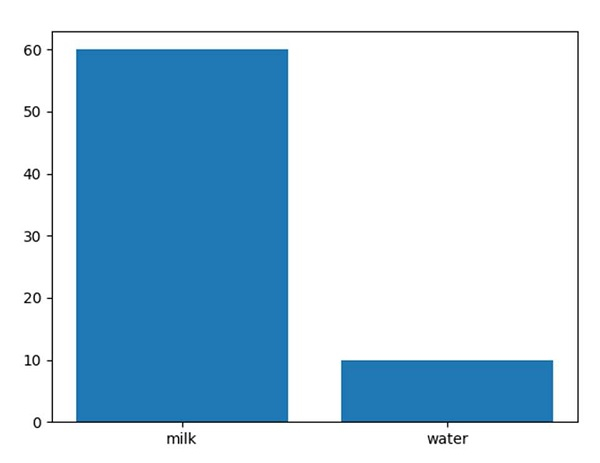# Plot a bar using matplotlib using a dictionary

First, we can define our dictionary and then, convert that dictionary into keys and values. Finally, we can use the data to plot a bar chart.

## Steps

• Create a dictionary, i.e., data, where milk and water are the keys.

• Get the list of keys of the dictionary.

• Get the list of values of the dictionary.

• Plot the bar using plt.bar().

• Using plt.show(), show the figure.

## Example

import matplotlib.pyplot as plt

data = {'milk': 60, 'water': 10}
names = list(data.keys())
values = list(data.values())

plt.bar(range(len(data)), values, tick_label=names)
plt.show()

## Output